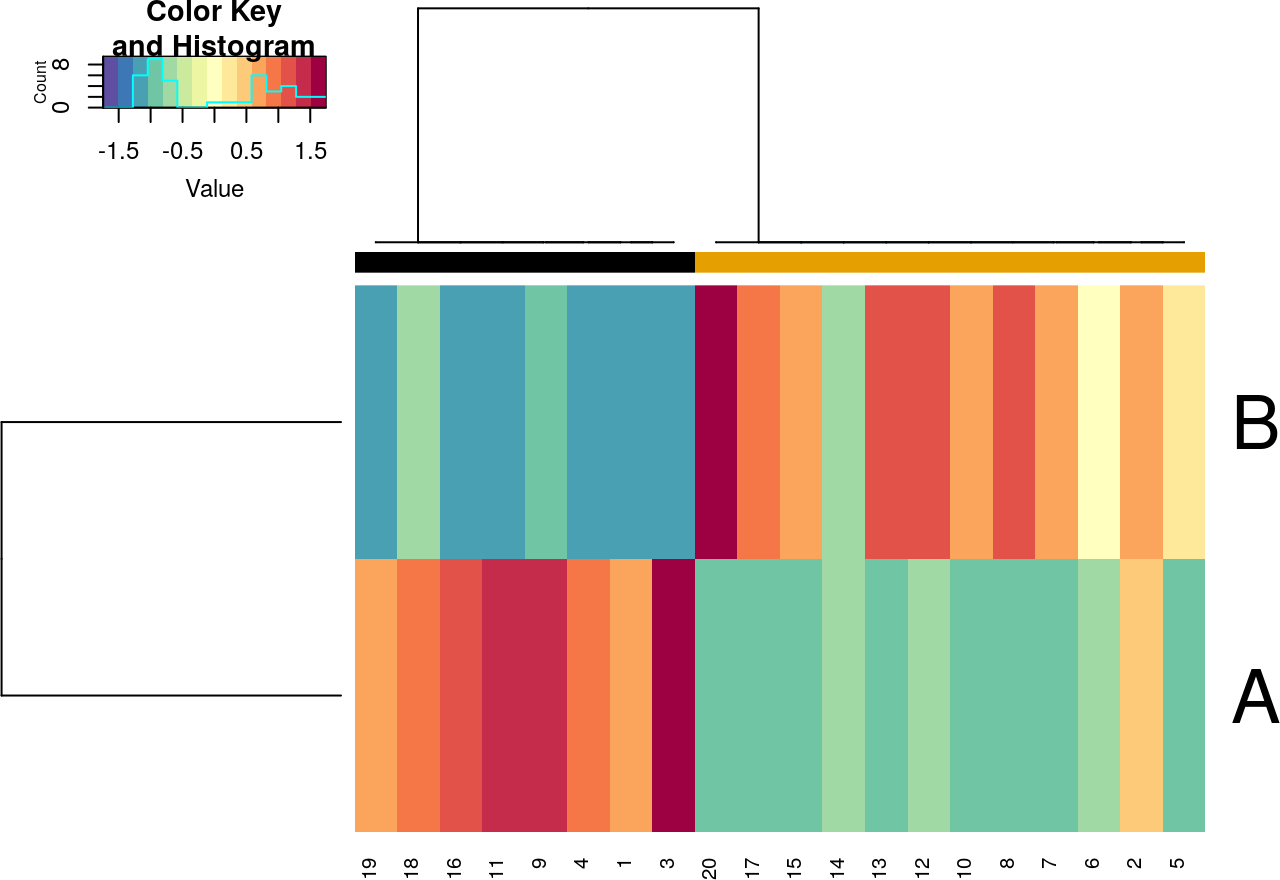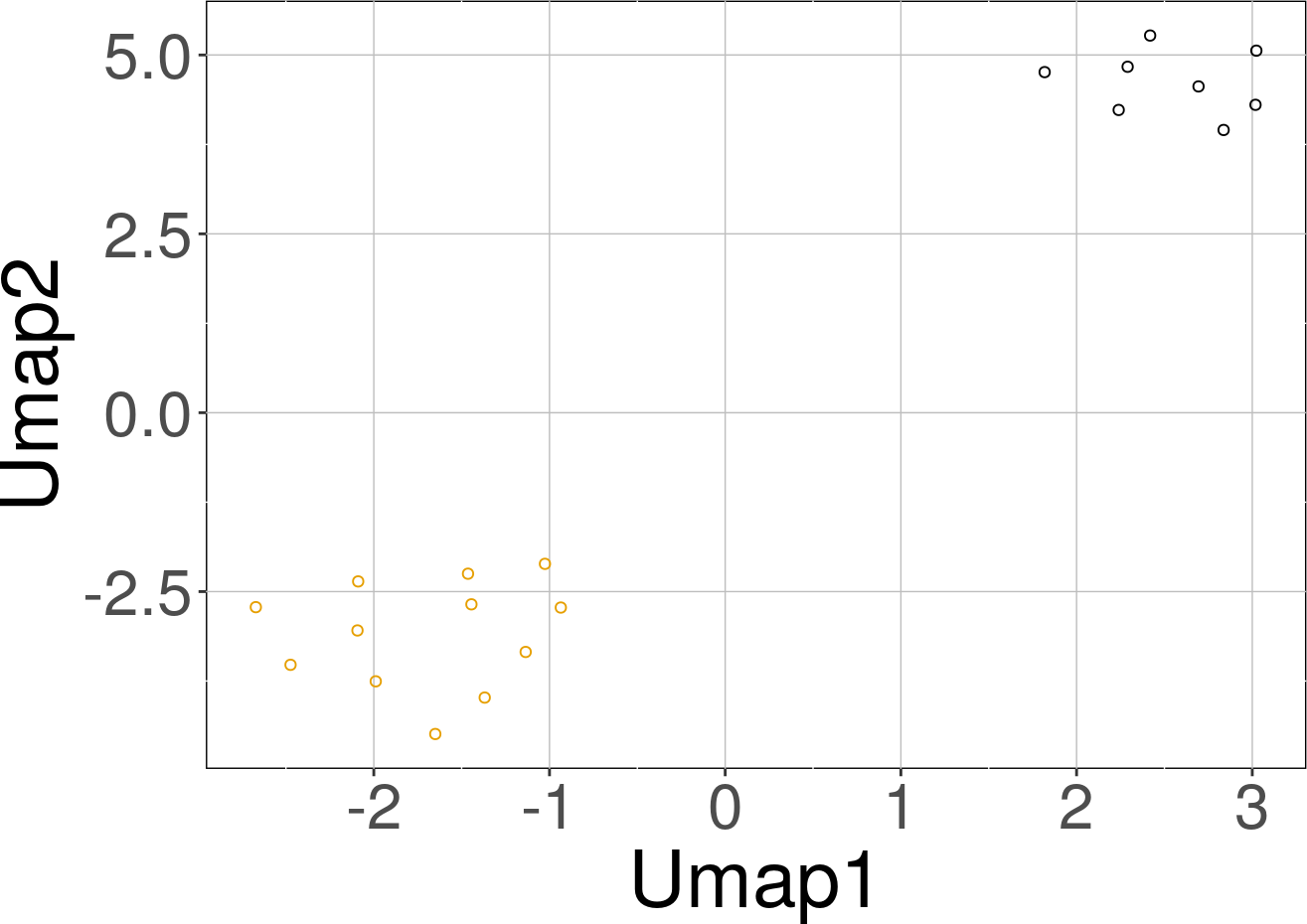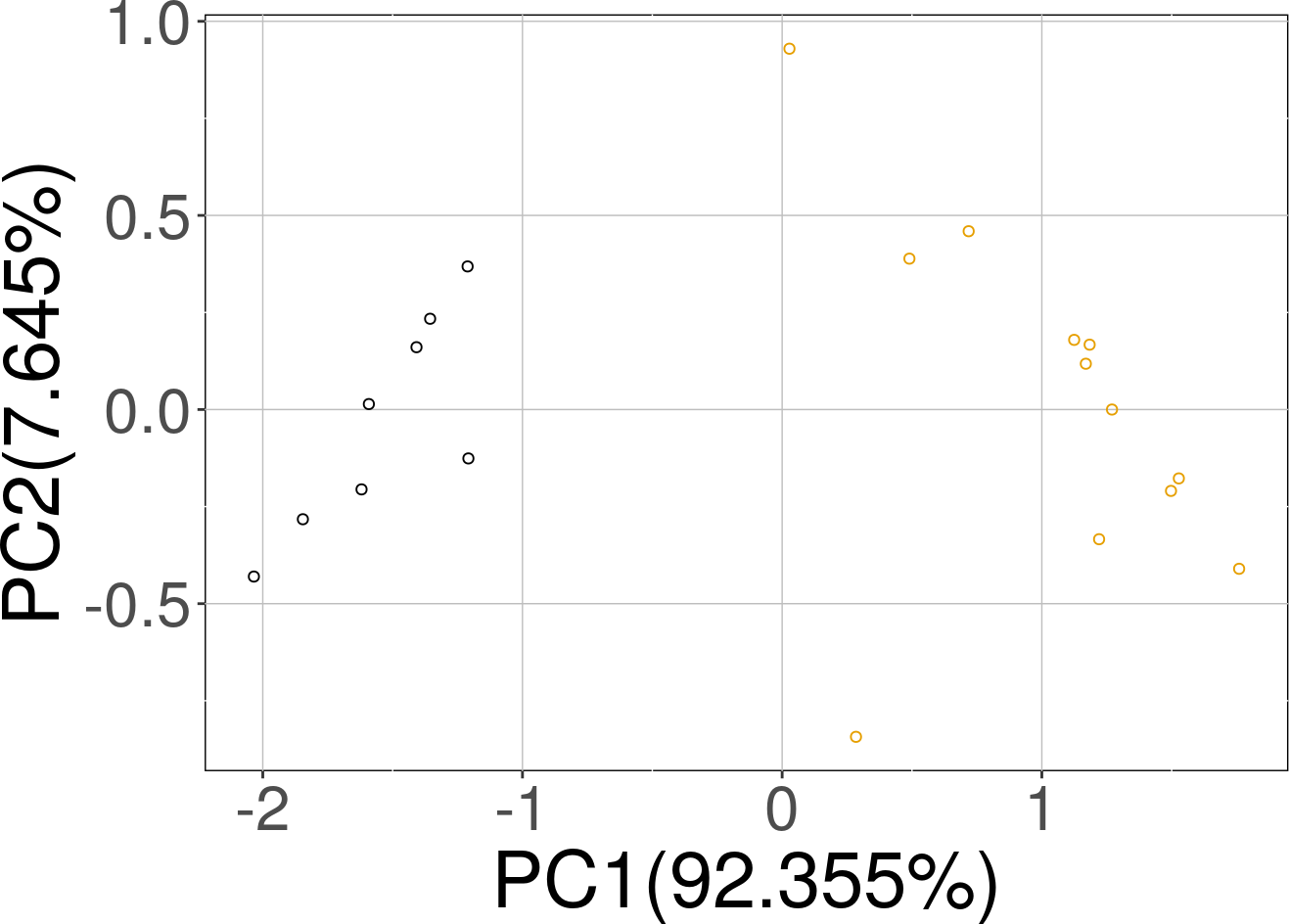# 1 Introduction

This document illustrates the use of sRACIPE to simulate any circuit or network or topology (used interchangeably) and analyze the generated data. sRACIPE implements a randomization-based method for gene circuit modeling. It allows us to study the effect of both the gene expression noise and the parametric variation on any gene regulatory circuit (GRC) using only its topology, and simulates an ensemble of models with random kinetic parameters at multiple noise levels. Statistical analysis of the generated gene expressions reveals the basin of attraction and stability of various phenotypic states and their changes associated with intrinsic and extrinsic noises. sRACIPE provides a holistic picture to evaluate the effects of both the stochastic nature of cellular processes and the parametric variation.

## 1.1 Installation

``````if (!requireNamespace("BiocManager", quietly = TRUE))
install.packages("BiocManager")
BiocManager::install("sRACIPE")``````

Or install the development version of the package.

``BiocManager::install(“vivekkohar/sRACIPE”)``
1. Load the package into R session.
``library(sRACIPE)``

For simplicity, we will start with the toggle switch with mutual inhibition and self activation of both genes as a test case. Additionally, to keep the simulations fast, we will use fewer models, small integration time and longer integration step size. We recommend using the default settings for most parameters for actual simulations.

One can use the topology stored in a text file or loaded as a dataframe. The typical format of the topology file is a 3 column file where the first column is name of the source gene, second column is name of the target gene and last column is the interaction type (1 - activation, 2-inhibition). The first line should contain the header (Source Target Interaction). We will work with a demo circuit from the package.

``````library(sRACIPE)

data("demoCircuit")
demoCircuit``````
``````##   Source Target Type
## 1      A      B    2
## 2      B      A    2
## 3      A      A    1
## 4      B      B    1``````

# 3 Simulate the circuit

We will use a reduced number of models (using numModels) for demonstration. The simulations will return a RacipeSE object.

``````rSet <- sRACIPE::sracipeSimulate(circuit = demoCircuit, numModels = 20,
plots = FALSE, integrateStepSize = 0.1,
simulationTime = 30)``````
``## circuit file successfully loaded``
``## Generating gene thresholds``
``## generating thresholds for uniform distribution1...``
``## Running the simulations``
``## ========================================``

# 4 Plotting the simulated data

We can plot the simulated data using the sracipePlotData function or using plots=TRUE in sracipeSimulate. The data can be normalized before plotting. Otherwise it will be normalized by the plotting function. By default, two clusters are identified and models colored according to hierarchical clustering.

``````rSet <- sRACIPE::sracipeNormalize(rSet)
rSet <- sRACIPE::sracipePlotData(rSet, plotToFile = FALSE)``````# 5 Knockdown Analysis

The simulations can be used to perform in-silico perturbation analysis. For example, here we will limit a gene’s production rate to mimic its knockdown and show how that changes the relative proportion of models in different clusters.

``````data("demoCircuit")
rSet <- sRACIPE::sracipeSimulate(circuit = demoCircuit,
numModels = 50, plots = FALSE,
integrateStepSize = 0.1,
simulationTime = 30)``````
``## circuit file successfully loaded``
``## Generating gene thresholds``
``## generating thresholds for uniform distribution1...``
``## Running the simulations``
``## ========================================``
``````kd <- sRACIPE::sracipeKnockDown(rSet, plotToFile = FALSE,
reduceProduction=50)``````